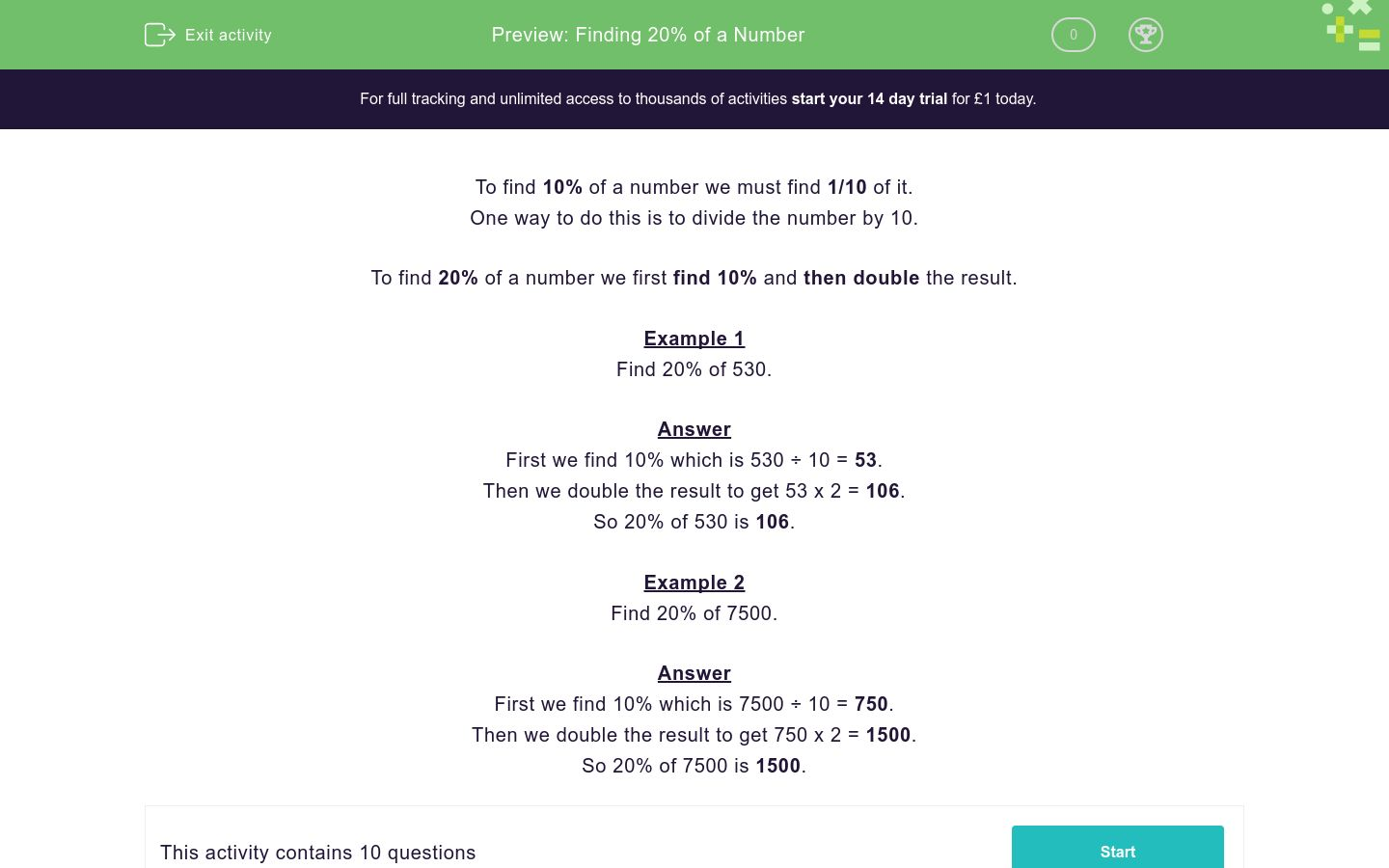# Finding 20% of a Number

In this worksheet, students must find 20% of the given numbers.Key stage:  KS 2

Curriculum topic:  Ratio and Proportion

Curriculum subtopic:  Solve Percentage Problems

Difficulty level:### QUESTION 1 of 10

To find 10% of a number we must find 1/10 of it.

One way to do this is to divide the number by 10.

To find 20% of a number we first find 10% and then double the result.

Example 1

Find 20% of 530.

First we find 10% which is 530 ÷ 10 = 53.

Then we double the result to get 53 x 2 = 106.

So 20% of 530 is 106.

Example 2

Find 20% of 7500.

First we find 10% which is 7500 ÷ 10 = 750.

Then we double the result to get 750 x 2 = 1500.

So 20% of 7500 is 1500.

Work out:

20% of 540

Work out:

20% of 50

Work out:

20% of 90

Work out:

20% of 910

Work out:

20% of 460

Work out:

20% of 120

Work out:

20% of 220

Work out:

20% of 720

Work out:

20% of 1200

Work out:

20% of 3100

• Question 1

Work out:

20% of 540

108
EDDIE SAYS
540 ÷ 10 = 54
54 × 2 = 108
• Question 2

Work out:

20% of 50

10
EDDIE SAYS
50 ÷ 10 = 5
5 × 2 = 10
• Question 3

Work out:

20% of 90

18
EDDIE SAYS
90 ÷ 10 = 9
9 × 2 = 18
• Question 4

Work out:

20% of 910

182
EDDIE SAYS
910 ÷ 10 = 91
91 × 2 = 182
• Question 5

Work out:

20% of 460

92
EDDIE SAYS
460 ÷ 10 = 46
46 × 2 = 92
• Question 6

Work out:

20% of 120

24
EDDIE SAYS
120 ÷ 10 = 12
12 × 2 = 24
• Question 7

Work out:

20% of 220

44
EDDIE SAYS
220 ÷ 10 = 22
22 × 2 = 44
• Question 8

Work out:

20% of 720

144
EDDIE SAYS
720 ÷ 10 = 72
72 × 2 = 144
• Question 9

Work out:

20% of 1200

240
EDDIE SAYS
1200 ÷ 10 = 120
120 × 2 = 240
• Question 10

Work out:

20% of 3100